#### Filter Circuits, Physics tutorial

Filter Circuits:

The main function of filter circuit is to minimize ripple content in rectifier output. As seen, output of different rectifier circuits is pulsating. It has dc value and some ac components known as ripples. This kind of output is not helpful for driving sophisticated electronic circuits/ devices. In fact, these circuits need a very steady dc output which approaches smoothness of battery's output.

The circuit which converts pulsating output from rectifier into a very steady dc level is called as filter as it filters out or smoothens out pulsations in output. Consider given popular filter circuits:

1. Shunt capacitor filter

2. Series inductor filter

3. L-C filter (or L-type)

4. R-C filter

5. C-L-C filter.

Shunt Capacitor Filter:

In this circuit, suitable single capacitor C is connected across rectifier and in parallel with load RL to get filtering action. This kind of filter is called as capacitor input filter.

This filter circuit depends for its operation on property of the capacitor to charge up (i.e. store energy) during conducting half-cycle and to discharge (i.e. deliver energy) during non-conducting half-cycle. Capacitor opposes any change in voltage. When connected across pulsating dc. Voltage, it tends to smoothen out or filter out voltage pulsations (or ripples). Filtering action of simple capacitor filter when used in half-wave rectifier can be understood.

Circuit Analysis:

When positive half-cycle of ac input is applied, diode is forward-biased and therefore is turned ON. This permits C to quickly charge up to peak value of input voltage Vip because charging time constant is almost zero. It is so because there is no resistance in charging path except diode forward resistance that is negligible. Therefore, capacitor follows charging. After being fully charged, capacitor holds charge till input ac supply to rectifier goes negative. During negative half-cycle, capacitor tries to discharge. Though, it can't discharge through diode that, being now reverse-biased, is OFF. Therefore, it discharges through RL from point b to c and its voltage reduces somewhat. Discharging time constant (=CRL) is usually 100 times more than charging time. Therefore, C doesn't have sufficient time to discharge noticeably.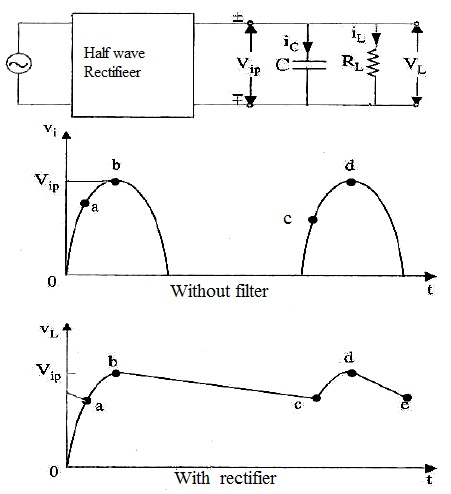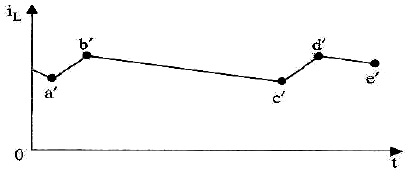During next positive half-cycle, when rectifier voltage exceeds capacitor voltage represented by point C, C is again charged quickly to Vip as represented by point d. Once more, input voltage goes negative, opening diode and forcing C to discharge through RL during interval de. In this way, RL sees a nearly constant dc voltage across it at all times.

The filtering action of this simple capacitor filter on a full-wave rectifier is shown. It is seen that as compared to half-wave rectifier. dc load voltage increases slightly towards Vip, Ripple voltage has been reduced by half. Decreased ripple is due to shorter discharge time before capacitor is re-energized by another pulse of current.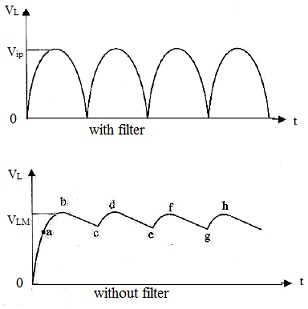Load current has same wave-shape as vL as load is purely resistive. During periods a'b' and c'd' etc., current is supplied by diode and during periods b'c' and d'e' etc. by capacitor.

Diode Current:

Diode current flows during short intervals of time like ab and cd etc that is reproduced. During these intervals, diode output voltage is greater than capacitor voltage that is also load voltage. Therefore, diode current is surging current i.e. it takes form of short-duration pulses. Small resistor is always joined in series with diode to limit surge current. It is called as surge limiting resistor. Sole function of diode is to recharge and sole function of is to supply load current by discharge.

Effect of increasing filter capacitance:

The capacitor has basic property of opposing changes in voltage. Therefore, bigger capacitor would tend to decrease ripple magnitude. It has been found that increasing capacitor size.

1. Increases Vdc towards limiting value Vip;

2. Reduces magnitude of ripple voltage;

3. Reduces the time of flow of current pulse through the diode;

4. Increases peak current in diode.

Calculations of shunt capacitor filter: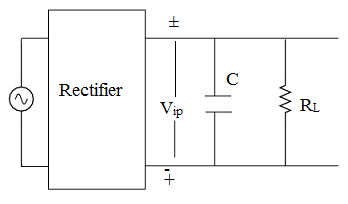Consider rectifier and filter circuit where capacitor has been connected across. Output voltage waveforms of half-wave rectifier with the shunt capacitor filter while another shows those for full-wave rectifier. Ripple voltage that occurs under light load conditions can be approximated by the triangular wave that has peak-to-peak value of Vr(p-p) and time period of Tr (as charging time is negligibly small, approximate discharging time represents full time-period.) centered around dc level.

In fact, Vr(p-p) is amount by which capacitor voltage falls during discharge period Tr. This discharge is really exponential (given by V0 = Vipe-t/CRL) but can be approximated by the straight line discharge if we suppose discharge rate to remain constant at dc level Idc. In that case, charge lost dQ in time Tr is IdcTr

Idc = Vdc/RL

Triangle ripple has the rms value given by:

Vr(rms) = Vr(p-p)/2/√3 = Vdc/2√3 frCRL

Therefore γ = Vr(rms/Vdc) = 1/2√3 frCRL

Now, fr is frequency of ripple voltage. For the half-wave rectifier fr, equals rectifier line input frequency while it is double line input frequency for the full-wave rectifier. If f is line frequency, then

γ ≈ 1/2√3 fCRL for half wave rectifier

≈ 1/4√3 fCRL for full wave rectifier

Series inductor filter:

The filter consists of the choke in series with load resistor. Operation of such a filter depends on fundamental property of the inductor to oppose any sudden changes in current flowing through it. As this inductor presents high impedance to ac components in filter output, it decreases their amplitude with respect to dc component thereby producing only small ripple. Fourier series for rectifier output voltage is

vi = Vip(2/π - 4/3π cos2ωt - 4/15πcos4ωt - .....)

For finding ripple factor, compute the dc as well as ac drop over RL. If we neglect choke resistance (RC) then the entire dc component of filter output is available across RL and its value is Vdc - 2Vip/π.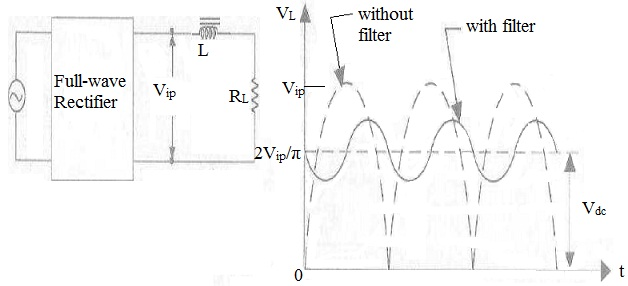Consider only the second harmonic voltage (4Vip/3π)cos2ωt of 2ω frequency and neglect higher harmonic voltages. This ac voltage partly drops over XL and partly over RL. Since choke and RL are connected in series, the maximum value of drop over RL is

=(4Vip/3π)(RL/√R2L + X2L)

The rms value of this ac voltage drop across RL is

Vac = (4Vip/√2.3π)(RL/√R2L + X2L) γ = Vac/Vdc = (4Vip/√2.3π)(RL/√R2L + X2L) X π/2Vip

γ = √2RL/3x2ωL

It is seen that ripple decreases as RL decreases or load current increases (just the opposite of what happens in the case of shunt capacitor filter).

The Choke Input or L-C Filter:

It is a combination of two filters and provides a lower ripple than is possible with either L or C alone. As is known, in an inductor filter, ripple increases with RL but decreases in a capacitor filter. The combination of L and C (i.e L-C-section) filter makes the ripple independent of RL.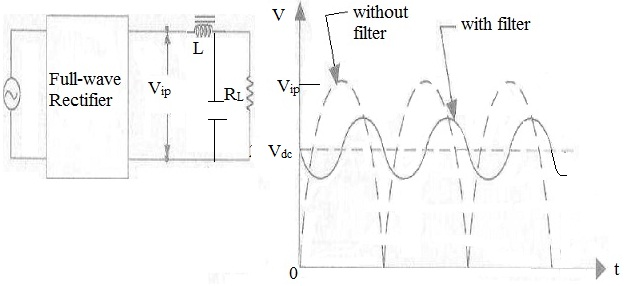Ripple Factor:

The ac drop over RL is the same as across C. As XC<<RL, parallel combination of RL and XC has impedance ≈XC. Second harmonic voltage (4Vip/3π)cos2ωt can be assumed to drop over L-C series combination as RL is effectively not there.

Maximum value of ac drop over C is

(4Vip/3π).(XC/(XL + XC))

γ = √2/(3(2ωC)(2ωL)) = √2/12ω2LC = 1.19/LC if C is in μF and L in henrys

The R-C Filter

Assume that it is joined to full-wave rectifier having a filtered output voltage of Vip. Dc component voltage that drops over RL is R-C Filter Circuit

(2Vip/π)(RL/(R + RL))

Again, we would consider only second harmonic voltage (4Vip/3π)cos2ωt. As before, it will be supposed that Xc<<RL so that RL||XC ≈ XC. In that case, ac voltage would be supposed to drop across R-C combination.

Vac = (1/√2)(4Vip/3π)(XC/√R2 + X2c) = (4Vip/√2.3π)(1/√1 + R2/X2C)

Same is drop across RL

γ = (√2/3)(1 + R/RL)/√1 + R2/X2C = (1 + R/RL)/(3√2ωCR) If R2/X2C>>1

The C-L-C or Pi Filter:

It has one inductor and two capacitors joined across its each end. Three components are arranged in shape of Greek letter π. It is also known as capacitor input π-filter. Input capacitor C1 is selected to offer very low reactance to ripple frequency. Therefore, major part of filtering is done by C1. Most of the remaining ripple is removed by combined action of L and C2. This circuit provides much better filtering than LC filter circuit. Though, C1 is still directly connected across supply and would require high pulses of current if load current is large. As these high peak current pulses are likely to damage rectifier diode, this filter is utilized with low-current equipment.

However this filter-gives rather higher output voltage, its voltage regulation is inferior to that of LC filter.

Ripple factor of this filter is provided by:

γ = √2XC1XC2/RLXL = √2/8ω3C1C2LRL = 5700/LC1C2RL when f = 50Hz

Here C1C2 are in μF, L in henrys and RL in ohms.

Bleeder Resistor:

Very often, resistor (known as bleeder resistor) is placed across filter output as it gives the following advantages:

1) It enhances voltage regulation of supply. By acting as the pre-load on supply, it causes initial voltage drop. When real load is connected, there so only a small amount of additional drop. In this way, difference between no-load and full-load voltage is decreased thereby enhancing regulation.

2) It gives safety to technicians handling equipment. When power supply is switched off, it gives a path for filter capacitor to discharge through. That is why it is known as bleeder resistor. Without it, capacitor will keep its charge for quite some time even when power supply is switched off. High voltage can be dangerous for people working with equipment.

3) By maintaining minimum current through choke, it enhances filtering action. Value of RE must be such as to conduct 10% of total load current.

Voltage Dividers:

Often more than one dc voltage is required for operation of electronic circuits. The single power supply can give as many voltages as are required by using voltage divider. Voltage divider is single tapped resistor joined across output terminals of supply. Tapped resistor may comprise of two or three resistors joined in series across supply. In fact, bleeder resistor may also be utilized as voltage divider.

Complete Power Supply:

It comprises of transformer with current-limiting resistor R1, rectifier diodes for full-wave rectification, a π-type filter, transistor series voltage regulator and a voltage divider. As seen, unregulated ac voltage is fed from transformer through full-wave rectifier. It is then filtered by CLC filter and lastly regulated by the transistor regulator. Regulated dc supply becomes available across voltage divider resistance RE. Output is practically ripple-free.

Troubleshooting Power Supplies:

There are generally two kinds of problems with power supplies i.e. either no dc output or low dc output. Situation of no dc output can take place because of any one of the given reasons:

1. When there is no output from rectifiers

2. When there is no ac input to power supply

3. When filter choke is open

4. When first input capacitor shorts.

A low dc output can happen in given situations:

1. Decreased input ac voltage

2. Open input capacitor of filter circuit

3. Partial short across load.

Tutorsglobe: A way to secure high grade in your curriculum (Online Tutoring)

Expand your confidence, grow study skills and improve your grades.

Since 2009, Tutorsglobe has proactively helped millions of students to get better grades in school, college or university and score well in competitive tests with live, one-on-one online tutoring.

Using an advanced developed tutoring system providing little or no wait time, the students are connected on-demand with a tutor at www.tutorsglobe.com. Students work one-on-one, in real-time with a tutor, communicating and studying using a virtual whiteboard technology.  Scientific and mathematical notation, symbols, geometric figures, graphing and freehand drawing can be rendered quickly and easily in the advanced whiteboard.

Free to know our price and packages for online physics tutoring. Chat with us or submit request at info@tutorsglobe.com